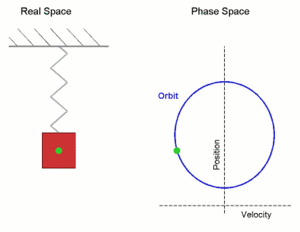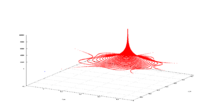# Orbit (dynamics)

In mathematics, in the study of dynamical systems, an orbit is a collection of points related by the evolution function of the dynamical system. The orbit is a subset of the phase space and the set of all orbits is a partition of the phase space, that is, different orbits do not intersect in the phase space. Understanding the properties of orbits by using topological methods is one of the objectives of the modern theory of dynamical systems.

For discrete-time dynamical systems, the orbits are sequences; for real dynamical systems, the orbits are curves; and for holomorphic dynamical systems, the orbits are Riemann surfaces.

## DefinitionDiagram showing the periodic orbit of a mass-spring system in simple harmonic motion. (Here the velocity and position axes have been reversed from the standard convention in order to align the two diagrams)

Given a dynamical system (T, M, Φ) with T a group, M a set and Φ the evolution functionwherewithwe definethen the setis called orbit through x. An orbit which consists of a single point is called constant orbit. A non-constant orbit is called closed or periodic if there exists ainsuch that.

### Real dynamical system

Given a real dynamical system (R, M, Φ), I(x) is an open interval in the real numbers, that is. For any x in Mis called positive semi-orbit through x andis called negative semi-orbit through x.

### Discrete time dynamical system

For discrete time dynamical system  :

forward orbit of x is a set :backward orbit of x is a set :and orbit of x is a set :where :

•is an evolution functionwhich is here an iterated function,
• setis dynamical space,
•is number of iteration, which is natural number and•is initial state of system andUsually different notation is used :

•is written as•whereisin the above notation.

### General dynamical system

For a general dynamical system, especially in homogeneous dynamics, when one has a "nice" groupacting on a probability spacein a measure-preserving way, an orbitwill be called periodic (or equivalently, closed) if the stabilizeris a lattice inside.

In addition, a related term is a bounded orbit, when the setis pre-compact inside.

The classification of orbits can lead to interesting questions with relations to other mathematical areas, for example the Oppenheim conjecture (proved by Margulis) and the Littlewood conjecture (partially proved by Lindenstrauss) are dealing with the question whether every bounded orbit of some natural action on the homogeneous spaceis indeed periodic one, this observation is due to Raghunathan and in different language due to Cassels and Swinnerton-Dyer . Such questions are intimately related to deep measure-classification theorems.

It is often the case that the evolution function can be understood to compose the elements of a group, in which case the group-theoretic orbits of the group action are the same thing as the dynamical orbits.

## ExamplesCritical orbit of discrete dynamical system based on complex quadratic polynomial. It tends to weakly attracting fixed point with multiplier=0.99993612384259

## Stability of orbits

A basic classification of orbits is

• constant orbits or fixed points
• periodic orbits
• non-constant and non-periodic orbits

An orbit can fail to be closed in two ways. It could be an asymptotically periodic orbit if it converges to a periodic orbit. Such orbits are not closed because they never truly repeat, but they become arbitrarily close to a repeating orbit. An orbit can also be chaotic. These orbits come arbitrarily close to the initial point, but fail to ever converge to a periodic orbit. They exhibit sensitive dependence on initial conditions, meaning that small differences in the initial value will cause large differences in future points of the orbit.

There are other properties of orbits that allow for different classifications. An orbit can be hyperbolic if nearby points approach or diverge from the orbit exponentially fast.